# Stata波动率专题：历史波动率、预测波动率、隐含波动率

Stata连享会   主页 || 视频 || 推文 || 知乎

New！ `lianxh` 命令发布了：

`. ssc install lianxh`

`. help lianxh`

⛳ Stata 系列推文：

## 2. 从随机游走到波动率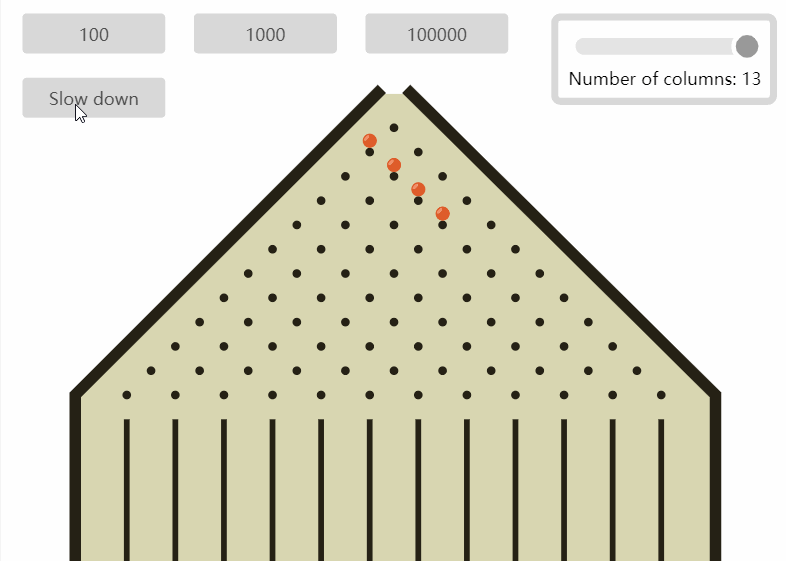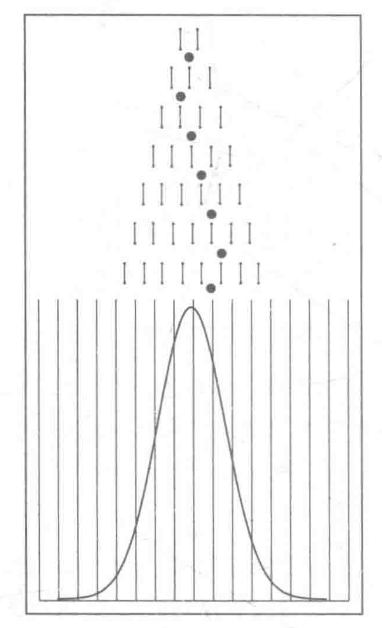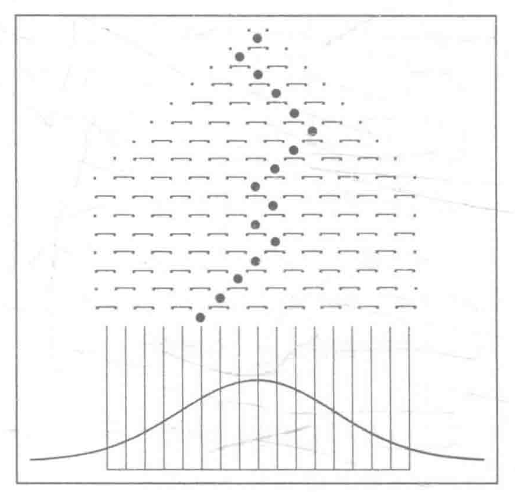``````. twoway function y=normalden(x), range(-5 5)  ///
|| function y=normalden(x, 0, 1.5),        ///
range(-5 5) lpattern(dash)            ///
|| function y=normalden(x, 0, 0.7),        ///
range(-5 5) lpattern(dash_dot)        ///
|| , title("不同波动率的正态分布")        ///
legend(ring(1) pos(6) cols(3)  ///
label(1 "中波动率")     ///
label(2 "高波动率")     ///
label(3 "低波动率"))    ///
scheme(s1color) note(资料来源：Stata连享会)
``````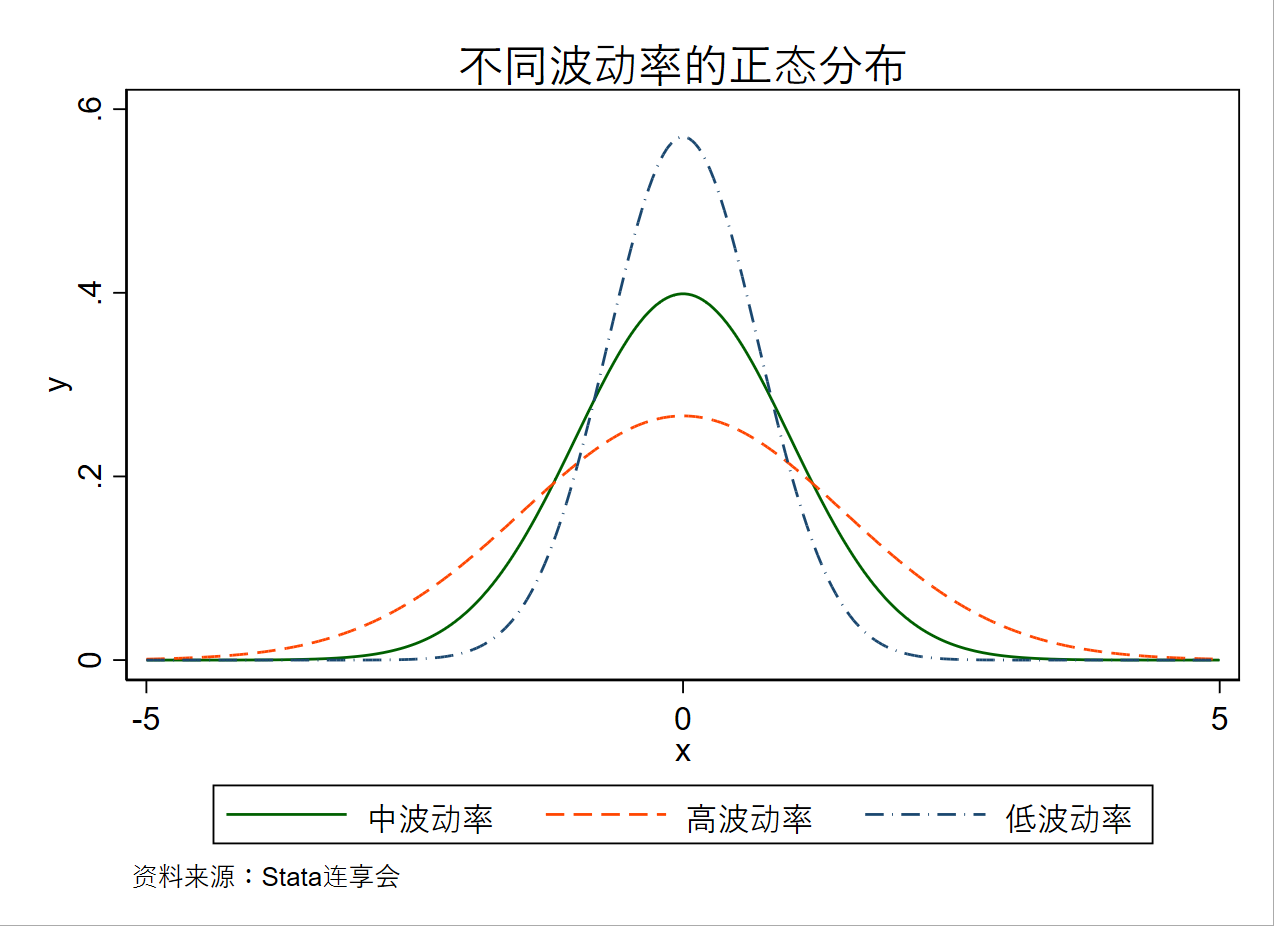## 3. 历史波动率

``````. ssc install cntrade, replace      // 安装 cntrade

. cntrade 300, index                // 提取沪深 300 指数数据

. global s 20210506
. gen t = _n
. gen return=clsprc/clsprc[_n-1]-1 //获取收益率
. rangestat (sd) return, interval(date -19 0)   //计算滚动标准差
. gen hv=return_sd*sqrt(252)                    //折算成为年化收益率
. drop if number<3
. rename hv 历史波动率
. keep if t>4300

. twoway (line 历史波动率 date, yaxis(1))  ///
(line clsprc date, yaxis(2) lpattern(dash)),  ///
scheme(s1color)   ///
legend(ring(1) pos(6)) ///
note(资料来源：Stata连享会)  ///
title("沪深300历史波动率(\$s)")
``````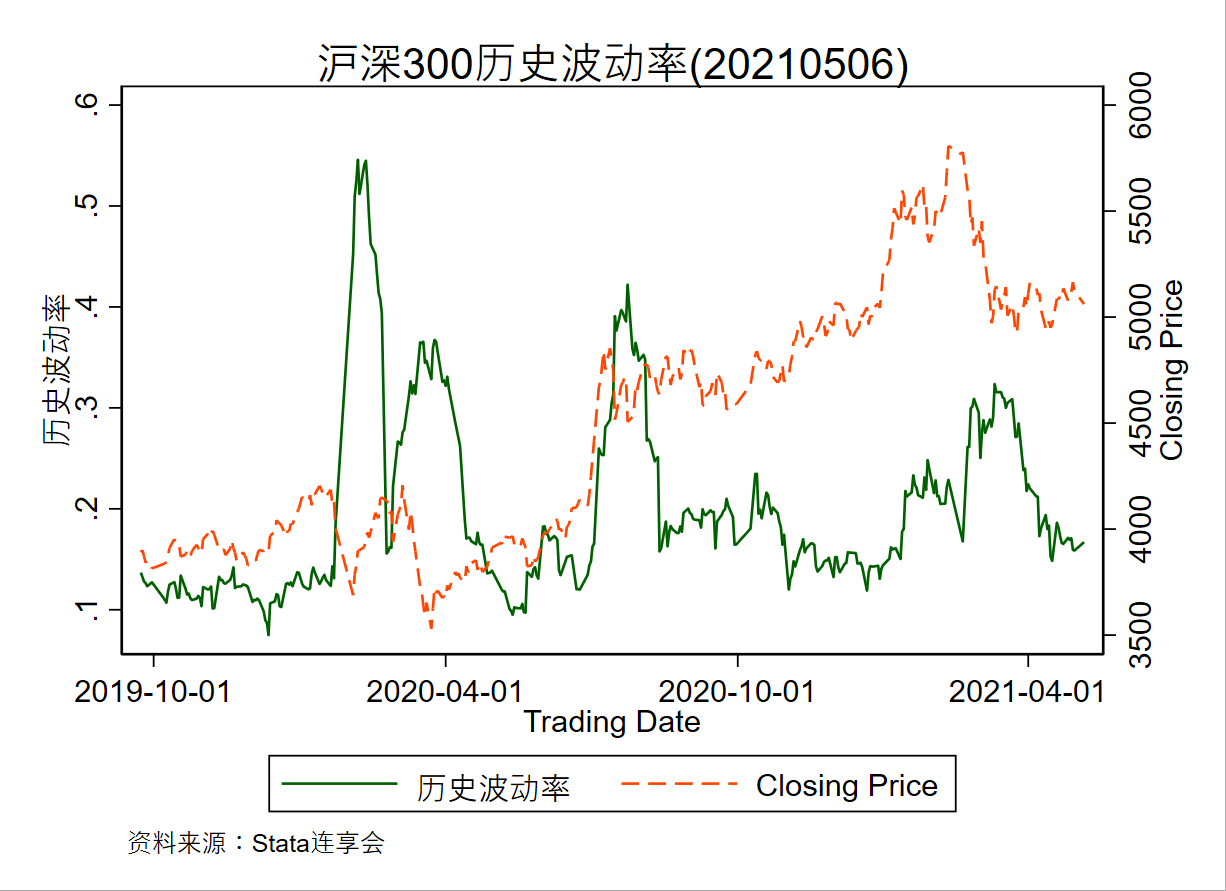``````. cntrade 300, index

. global s 20210506
. gen t = _n
. gen return=clsprc/clsprc[_n-1]-1
. gen return2=clsprc/clsprc[_n-1]-1
. gen return3=clsprc/clsprc[_n-1]-1

. rangestat (sd) return, interval(date -19 0)    // 滚动计算过去 20 日波动率

. gen hv20=return_sd*sqrt(252)
. rangestat (sd) return2, interval(date -59 0)   // 滚动计算过去 60 日波动率
. gen hv60=return2_sd*sqrt(252)

. rangestat (sd) return3, interval(date -119 0)  // 滚动计算过去 120 日波动率

. gen hv120=return3_sd*sqrt(252)
. label var hv20  "20日历史波动率"
. label var hv120 "120日历史波动率"
. label var hv60  "60日历史波动率"
. keep if t>4300

*-----------------graph-------begin----
#d ;
twoway (line hv20 date, yaxis(1))
(line hv60  date, yaxis(1) lpattern(dash_dot))
(line hv120 date, yaxis(1) lpattern(dash)),
scheme(s1color)
legend(ring(1) pos(6) col(3))
note(资料来源：Stata连享会)
title("沪深300不同时间段历史波动率(\$s)") ;
#d cr
*-----------------graph-------begin----
``````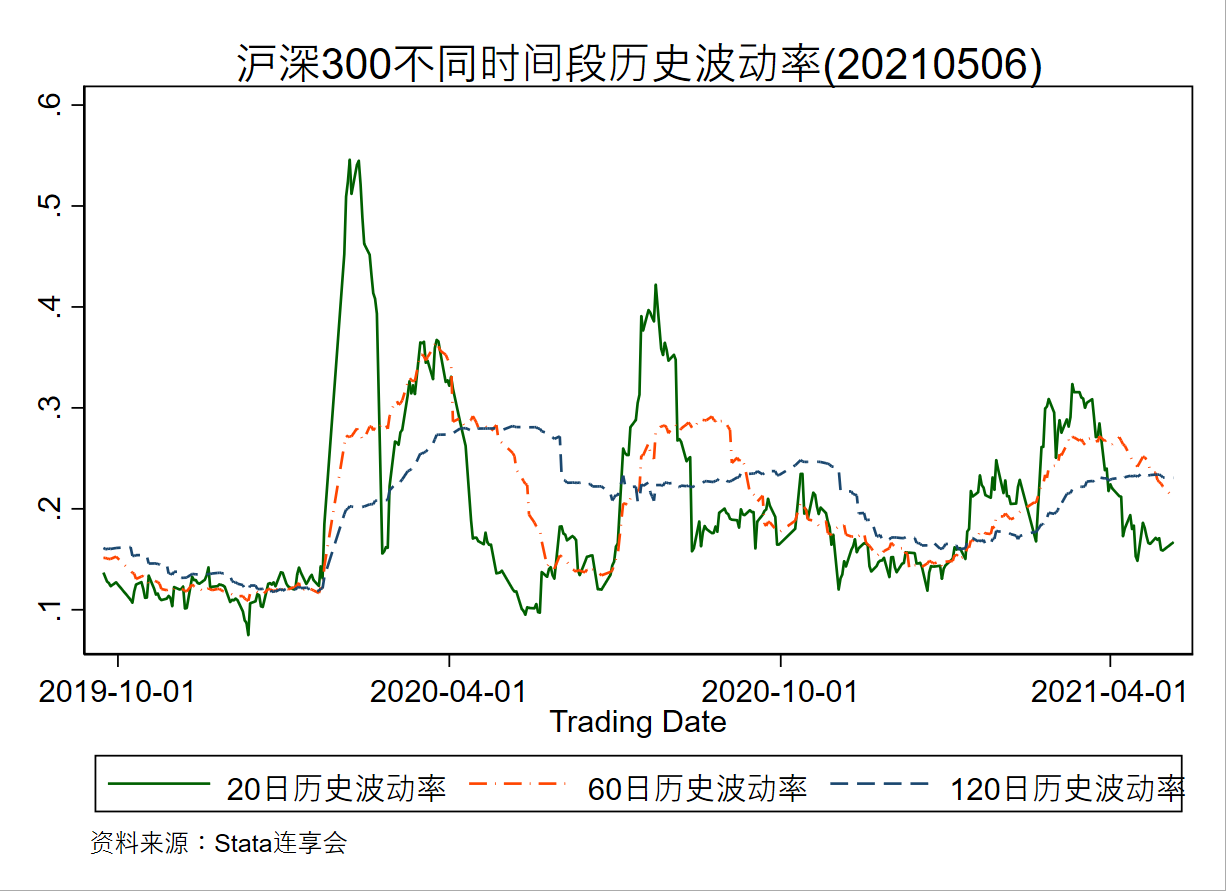## 4. 预测波动率

``````

. global s 20210506

. gen t = _n
. keep if t>3000
. gen return=clsprc/clsprc[_n-1]-1

. line return date, scheme(s1color)  title("沪深300收益率的时间趋势(\$s)") note(资料来源：Stata连享会)

. tsset t
. varsoc return, maxlag(8)    //用信息准则确定自回归模型的阶数
. var return, lags(1/7)
.   estimates store OLS

. predict e1, res
. g e2=e1^2

. ac  e2, scheme(s1color)    //画残差平方的自相关图
. pac e2, scheme(s1color)    //画残差平方的偏自相关图

. corrgram e2, lags(20)

. varsoc e2                                 //为了确定 p

. arch return L(1/7).return, arch(1/4) nolog          //ARCH(4)模型
.   estimates store ARCH4
. arch return L(1/7).return, arch(1) garch(1) nolog   //GARCH(1, 1)模型
.   estimates store GARCH11
. arch return L(1/7).return, arch(1) egarch(1) nolog  //EGARCH(1, 1)模型
.   estimates store EGARCH11
. arch return L(1/7).return, arch(1) garch(1) tarch(1) nolog  //TARCH(1)模型
.   estimates store TARCH1

//核密度图
. kdensity return, normopt(lpattern("-"))  ///
scheme(s1color) col(2)          ///
title("日收益率的核密度和正态密度") ///
note(资料来源：Stata连享会)

. quietly var return, lags(1/8)  //对扰动项的正态性进行统计检验
. varnorm
. arch return L(1/8).return, arch(1) garch(1) dist(t) nolog
.   estimates store GARCH11t
.   estimates table OLS ARCH4 GARCH11 EGARCH11 GARCH11t TARCH1   //对比不同方法
. quietly arch return L(1/8).return , arch(1) garch(1) nolog
. predict garchh, variance
. quietly arch return L(1/8).return, arch(1) dist(t) nolog
. predict archh, variance
. quietly arch return L(1/8).return, arch(1) egarch(1) nolog
. predict egarchh, variance                                     //预测条件方差
. keep if t>4500

*-绘图-----------------begin----
#d ;
. twoway (line archh date, yaxis(1))
(line garchh date, yaxis(1))
(line egarchh date, yaxis(1))
,
scheme(s1color)
legend(ring(1) cols(3) pos(6)
label(1 "ARCH(1)")
label(2 "GARCH(1, 1)")
label(3 "EGARCH(1, 1)"))
note(资料来源：Stata连享会)
title("沪深300预测波动率(\$s)");
*-绘图-----------------over-----
``````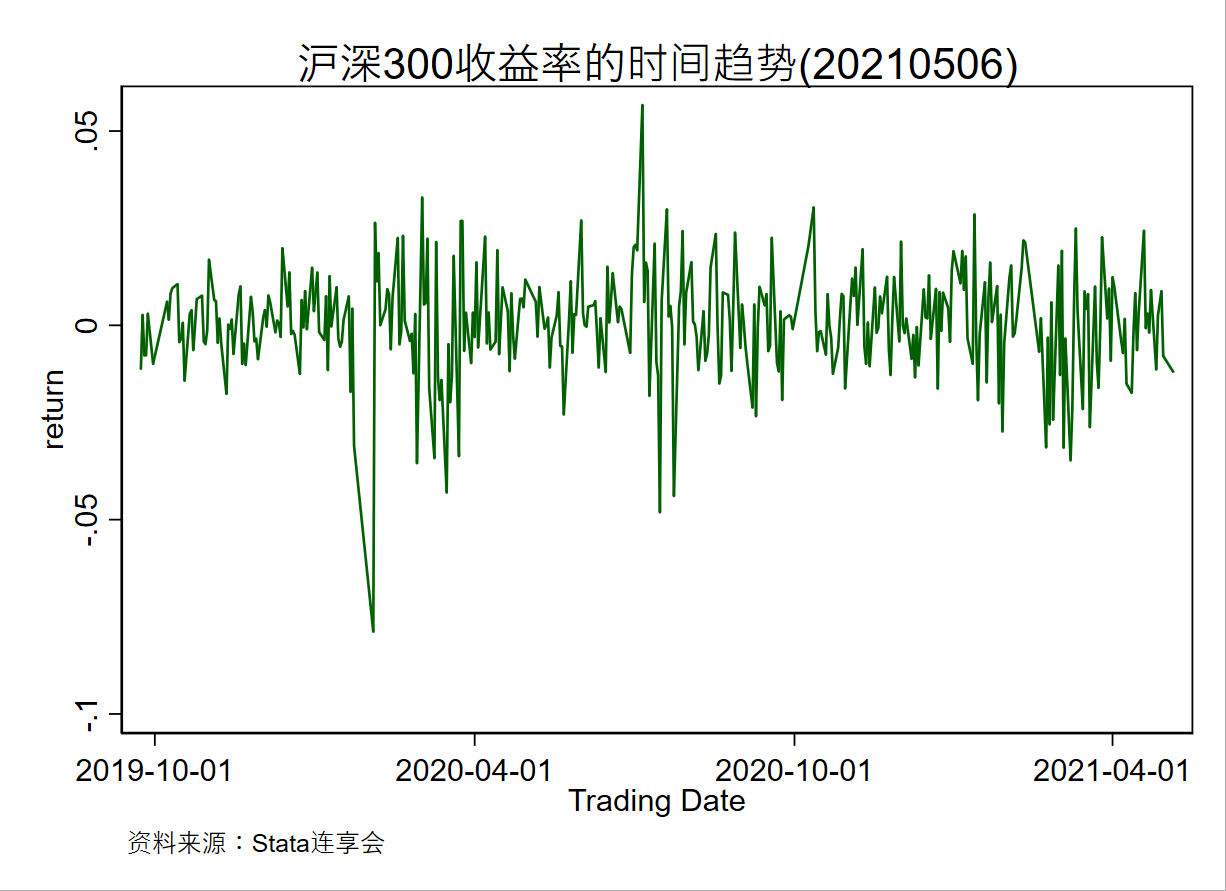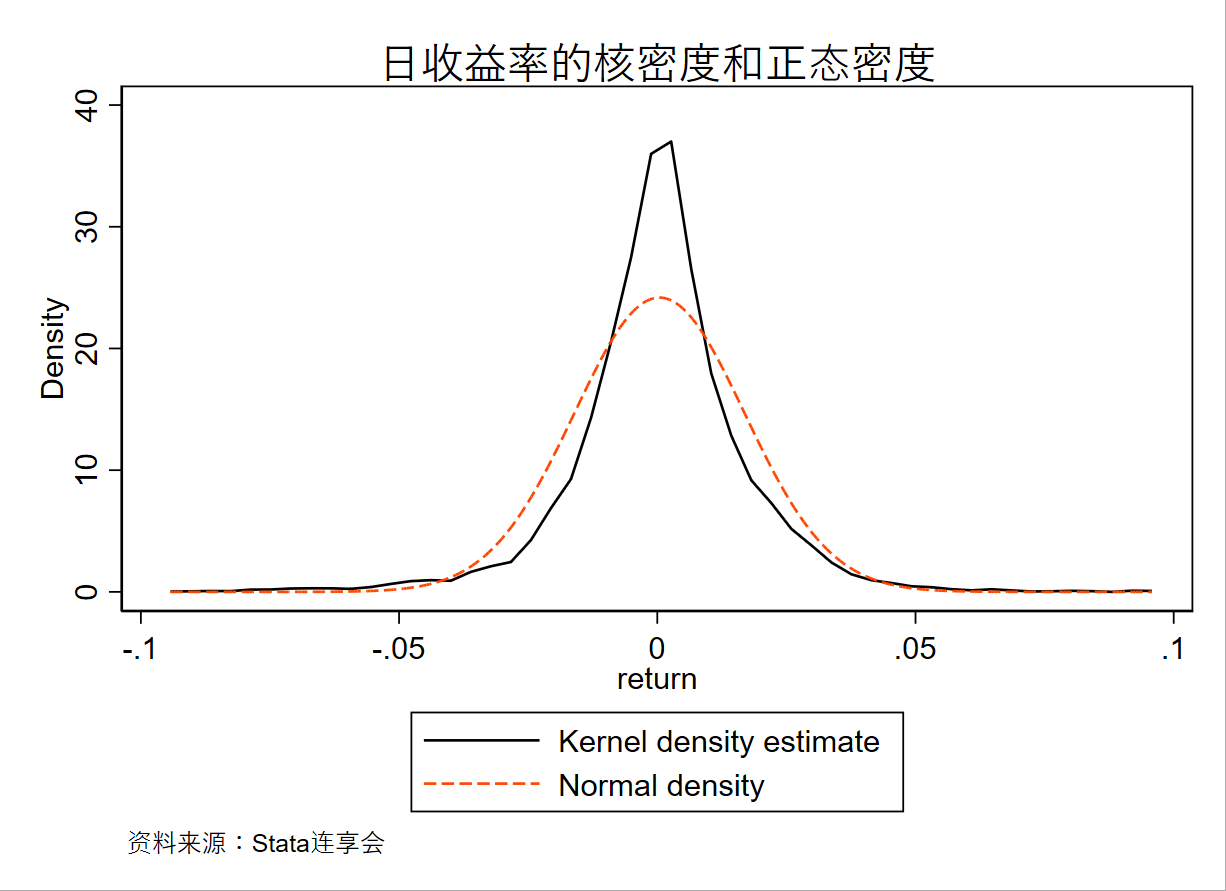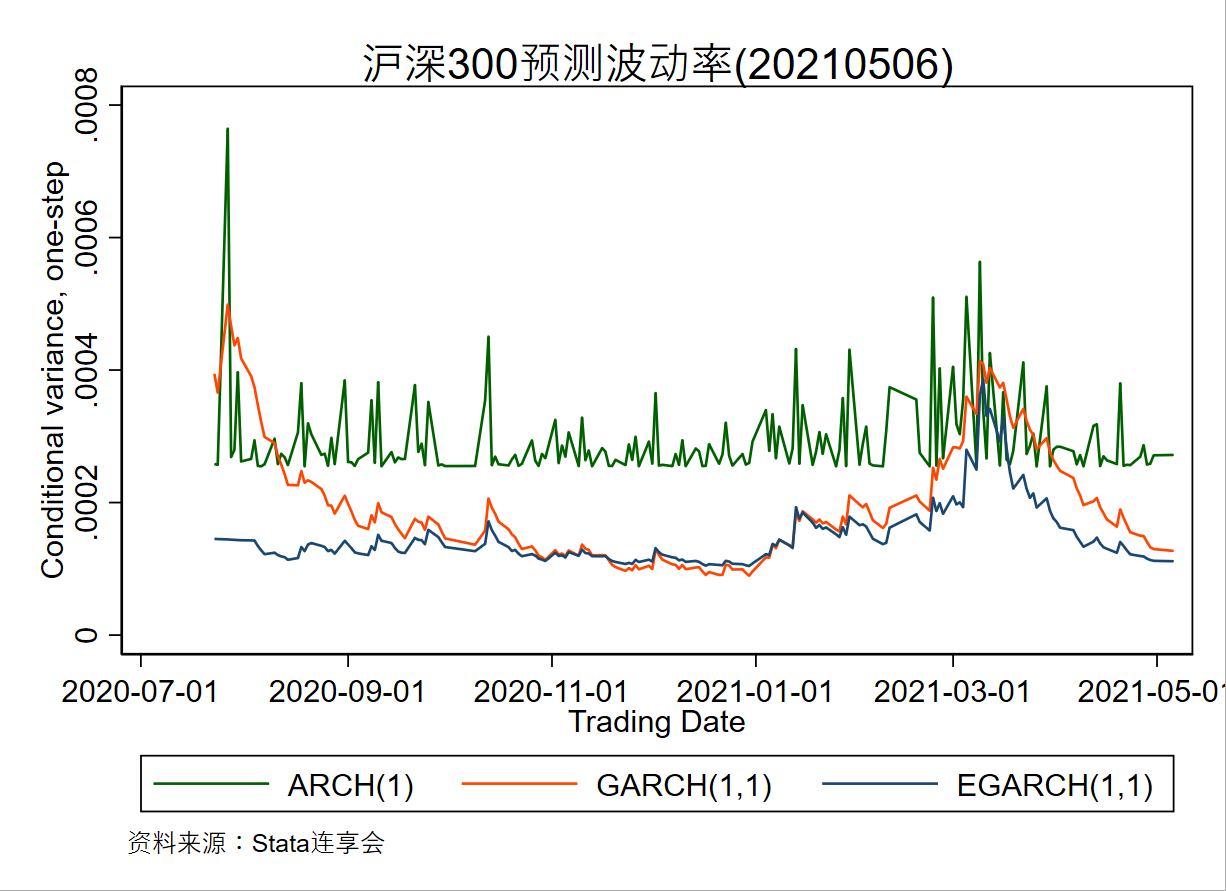## 5. 隐含波动率

``````real scalar bs(real scalar s0, real scalar k, real scalar t, real scalar r, real scalar d, real scalar sigma){
x=log(s0/k)+(r-d)*t
sig=sigma*sqrt(t)
d1=x/sig+sig/2
d2=d1-sig
pv=exp(-r*t)
result=s0*normal(d1)-pv*k*normal(d2)
return(result)
}
``````

``````real scalar hrimvol(real scalar s0, real scalar k, real scalar t, real scalar r, real scalar d, real scalar C){
sigmaL=1e-10
CL=bs(s0, k, t, r, d, sigmaL)
sigmaH=10
CH=bs(s0, k, t, r, d, sigmaH)
while (mean(sigmaH-sigmaL)>1e-10){
sigma=(sigmaL+sigmaH)/2
CM=bs(s0, k, t, r, d, sigma);
CL=CL+(CM<C)*(CM-CL)
sigmaL=sigmaL+(CM<C)*(sigma-sigmaL)
CH=CH+(CM>=C)*(CM-CH)
sigmaH=sigmaH+(CM>=C)*(sigma-sigmaH)
}
return(sigma)
}
``````

## 8. 相关推文

Note：产生如下推文列表的命令为：`lianxh Bootstrap 蒙特卡洛, m`

## 相关课程

### 最新课程-直播课

• Note: 部分课程的资料，PPT 等可以前往 连享会-直播课 主页查看，下载。

### 关于我们

• Stata连享会 由中山大学连玉君老师团队创办，定期分享实证分析经验。
• 连享会-主页知乎专栏，400+ 推文，实证分析不再抓狂。直播间 有很多视频课程，可以随时观看。
• 公众号关键词搜索/回复 功能已经上线。大家可以在公众号左下角点击键盘图标，输入简要关键词，以便快速呈现历史推文，获取工具软件和数据下载。常见关键词：`课程, 直播, 视频, 客服, 模型设定, 研究设计, stata, plus, 绘图, 编程, 面板, 论文重现, 可视化, RDD, DID, PSM, 合成控制法`

✏ 连享会-常见问题解答：
https://gitee.com/lianxh/Course/wikis

New！ `lianxh` 命令发布了：

`. ssc install lianxh`

`. help lianxh`